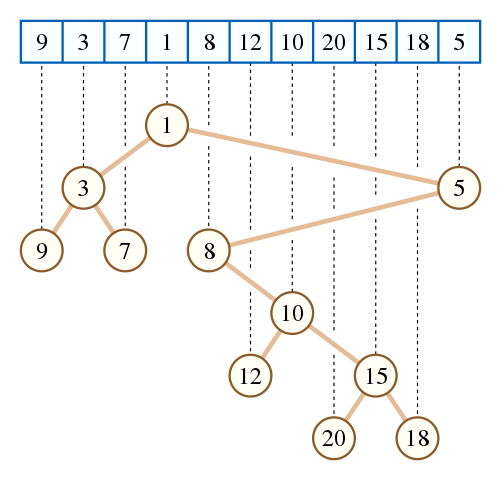# 思想

RT，该算法只适用于随机数据，如不随机会迅速退化。# 实现

Code:

int n;
int rt=0;
int l[MAXN*2],r[MAXN*2],a[MAXN*2];

int hsh(int x){
return (x*19260817)&((1<<20)-1);
}

int stk[MAXN*2];
void build(){
int top=0;
for(int i=0;i<n;i++){
int k=top;
while(k && (a[i]>a[stk[k]] || (a[i]==a[stk[k]] && hsh(i)>hsh(stk[k]))))k--;
if(k)r[stk[k]]=i;
if(k<top){
if(stk[k+1]==rt)rt=i;
l[i]=stk[k+1];
}
top=k+1;
stk[top]=i;
}
}
int query(int L,int R){
int x=rt;
while(x<L||x>R){
if(x<L)x=r[x];
else x=l[x];
}
return x;
}


# 复杂度证明

a<\frac{N}{2},b>\frac{N}{2}的概率为\frac{1}{2}\cdot\frac{1}{2}=\frac{1}{4}
a>\frac{N}{2},b<\frac{N}{2}的概率为\frac{1}{2}\cdot\frac{1}{2}=\frac{1}{4}

S=\sum_{i=1}^{\infty} \frac{i}{2^i}=\frac{1}{2}+\frac{2}{4}+\frac{3}{8}+\frac{4}{16}...

2S-S = 1+\frac{2}{2}-\frac{1}{2}+\frac{3}{4}-\frac{2}{4}... =\sum_{i=0}^{\infty} \frac{1}{2^i} = 2
S=2

TODO

---qwaszx的洛谷题解

# 带修玄学树/离散玄学树

THE END